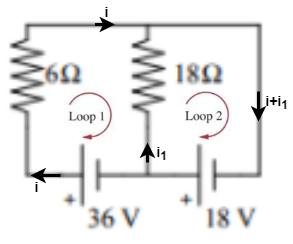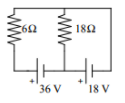# Problem: In the circuit shown, what is the current drawn from the 18 V battery? (1) 10.0 Α (2) 8.0 А (3)5.5 А (4) 4.5 А (5) 6.5 А

###### FREE Expert Solution

Kirchhoff's voltage law:

$\overline{){\mathbf{\Sigma }}{\mathbf{V}}{\mathbf{=}}{\mathbf{0}}}$

Also, remember that from Ohm's law:

$\overline{){\mathbf{V}}{\mathbf{=}}{\mathbf{i}}{\mathbf{R}}}$

Let's visualize the currents that flow in the circuit as follows:86% (247 ratings)###### Problem Details

In the circuit shown, what is the current drawn from the 18 V battery?(1) 10.0 Α

(2) 8.0 А

(3)5.5 А

(4) 4.5 А

(5) 6.5 А

Frequently Asked Questions

What scientific concept do you need to know in order to solve this problem?

Our tutors have indicated that to solve this problem you will need to apply the Kirchhoff's Loop Rule concept. You can view video lessons to learn Kirchhoff's Loop Rule. Or if you need more Kirchhoff's Loop Rule practice, you can also practice Kirchhoff's Loop Rule practice problems.

What professor is this problem relevant for?

Based on our data, we think this problem is relevant for Professor Werhner's class at UCF.